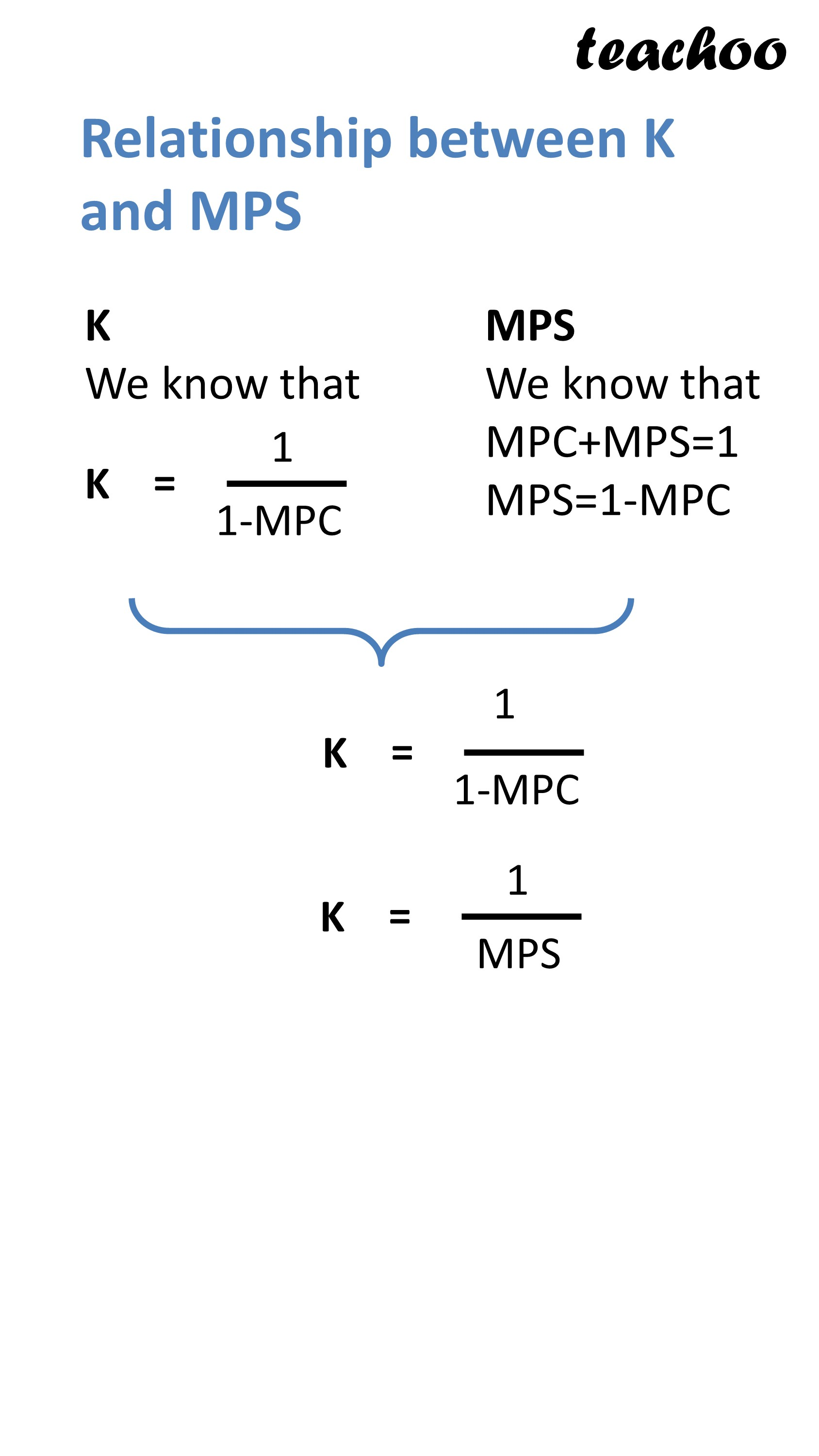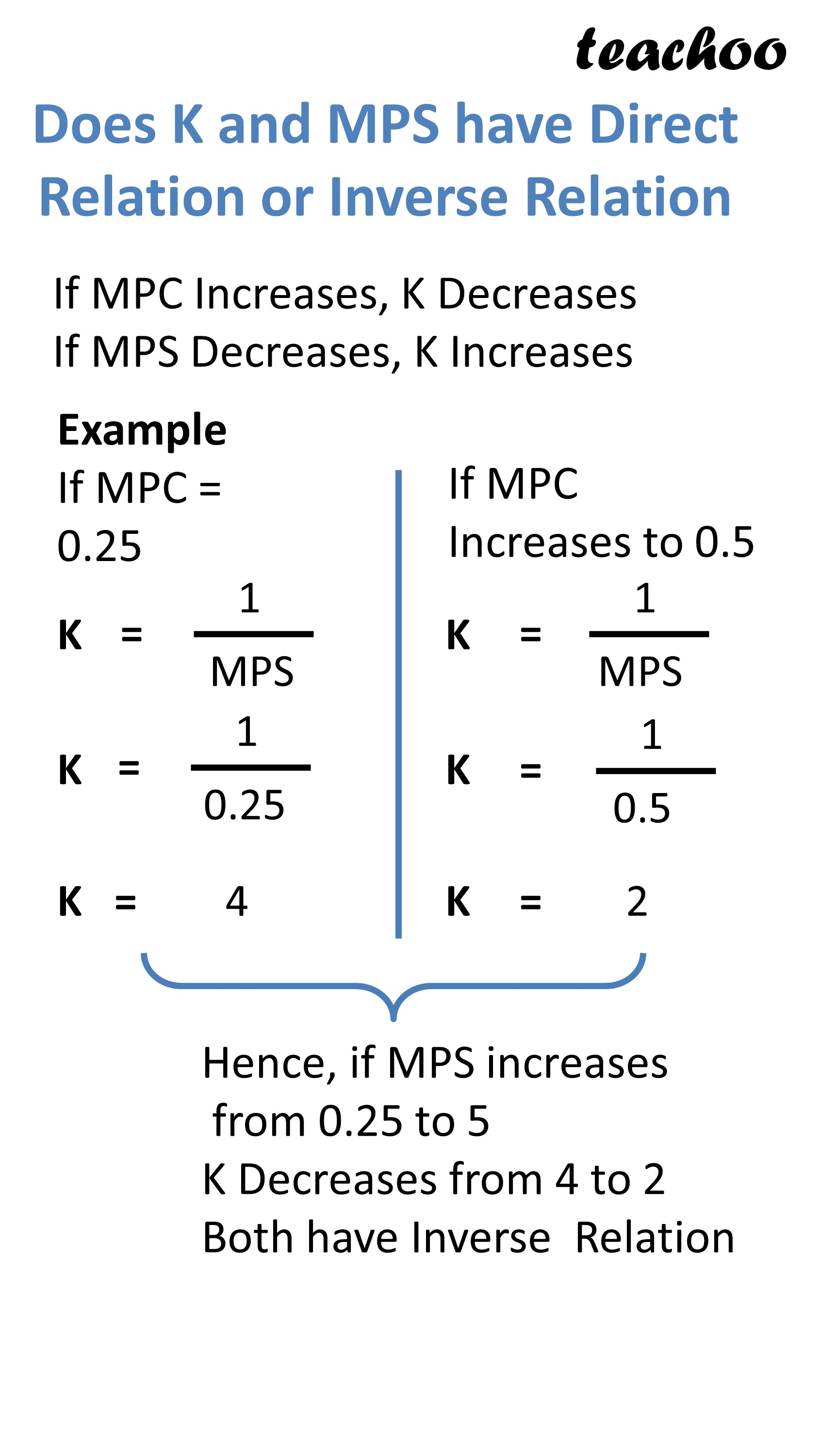Chapter 4 Part 2 - Income Determination and Multiplier

Economics Class 12
Macroeconomics

## Relation between Investment Multiplier k and MPS### Example 2.

Calculate the value of multiplier if the MPS is:

(a) 0.40;

(b) Equal to MPC

### Example 9.

Calculate MPS and Multiplier (k) from the following data:

 Income (Rs) 100 200 Saving (Rs) 40 100

### Example 6.

In an economy, the actual level of income is ₹ 500 crores, whereas, the full

employment level of income is ₹ 800 crores. If one-fourth of additional income is saved, calculate

increase in investment required to achieve full employment level of income.

### Example 8.

In an economy, the equilibrium level of income is ₹ 12,000 crore. The ratio of marginal propensity to consume and marginal propensity to save is 3:1. Calculate the additions investment needed to reach a new equilibrium level of income of ₹ 20,000 crore.

### Example 20.

In an economy, with every increase in income, 10 per cent of the rise in income is saved. Suppose a fresh investment of ₹ 120 crores takes place in the economy. Calculate the following:

(i) Change in the income;

(ii) Change in consumption.

### NCERT Questions

No questions in this part

### Other Books

#### Question 1

How is Investment Multiplier related to MPS?

#### Note

K and MPS have Inverse Relation

If MPC increases, K Decreases

IF MPS Decreases, K increases

#### Question 2

MPS = 0.25

Calculate value of Multiplier

Learn in your speed, with individual attention - Teachoo Maths 1-on-1 Class

### Transcript

Relationship between K and MPS K We know that MPS We know that MPC+MPS=1 MPS=1-MPC Does K and MPS have Direct Relation or Inverse Relation If MPC Increases, K Decreases If MPS Decreases, K Increases Example If MPC = 0.25 If MPC Increases to 0.5 Hence, if MPS increases from 0.25 to 5 K Decreases from 4 to 2 Both have Inverse Relation